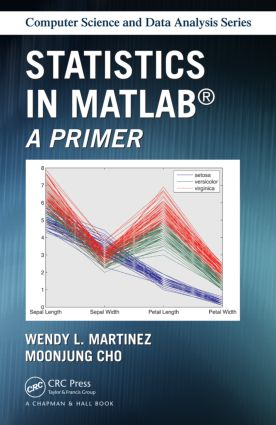# Statistics in MATLAB

## A Primer, 1st Edition

Chapman and Hall/CRC

286 pages | 76 B/W Illus.

##### Purchasing Options:\$ = USD
Paperback: 9781466596566
pub: 2014-12-15
\$54.95
x
Hardback: 9781138469310
pub: 2018-04-04
\$195.00
x
eBook (VitalSource) : 9780429167850
pub: 2014-12-15
from \$26.48

FREE Standard Shipping!

### Description

Fulfilling the need for a practical user’s guide, Statistics in MATLAB: A Primer provides an accessible introduction to the latest version of MATLAB® and its extensive functionality for statistics. Assuming a basic knowledge of statistics and probability as well as a fundamental understanding of linear algebra concepts, this book:

• Covers capabilities in the main MATLAB package, the Statistics Toolbox, and the student version of MATLAB
• Presents examples of how MATLAB can be used to analyze data
• Offers access to a companion website with data sets and additional examples
• Contains figures and visual aids to assist in application of the software
• Explains how to determine what method should be used for analysis

Statistics in MATLAB: A Primer is an ideal reference for undergraduate and graduate students in engineering, mathematics, statistics, economics, biostatistics, and computer science. It is also appropriate for a diverse professional market, making it a valuable addition to the libraries of researchers in statistics, computer science, data mining, machine learning, image analysis, signal processing, and engineering.

### Reviews

"The book provides an introductory but comprehensive guide for performing data analysis in MATLAB. It not only covers the most important topics in basic statistics (along with some machine learning techniques), but also touches upon more advanced methods such as kernel density estimation, bootstrap, and principal component analysis…Most of the theories are conveyed in a concise and intuitive way, yet the explanations are quite effective. The implementation of each method in MATLAB is demonstrated using real examples. Detailed MATLAB codes and corresponding numerical and figure outputs are presented with informative MATLAB comments, which makes them easily understood even without the context. The book can be used as a good complementary book to introductory statistics courses…The book can also serve as a perfect guide for self-learners who are not familiar with MATLAB but wish to use MATLAB as a data analysis tool."

—The American Statistician

List of Tables

Preface

MATLAB Basics

Desktop Environment

Getting Help and Other Documentation

Data Import and Export

Data I/O via the Command Line

The Import Wizard

Examples of Data I/O in MATLAB

Data I/O with the Statistics Toolbox

More Functions for Data I/O

Data in MATLAB

Data Objects in Base MATLAB

Accessing Data Elements

Examples of Joining Data Sets

Data Types in the Statistics Toolbox

Object–Oriented Programming

Miscellaneous Topics

File and Workspace Management

Punctuation in MATLAB

Arithmetic Operators

Functions in MATLAB

Summary and Further Reading

Visualizing Data

Basic Plot Functions

Plotting 2–D Data

Plotting 3–D Data

Examples

Scatter Plots

Basic 2–D and 3–D Scatter Plots

Scatter Plot Matrix

Examples

GUIs for Graphics

Simple Plot Editing

Plotting Tools Interface

PLOTS Tab

Summary and Further Reading

Descriptive Statistics

Measures of Location

Means, Medians, and Modes

Examples

Measures of Dispersion

Range

Variance and Standard Deviation

Covariance and Correlation

Examples

Describing the Distribution

Quantiles

Interquartile Range

Skewness

Examples

Visualizing the Data Distribution

Histograms

Probability Plots

Boxplots

Examples

Summary and Further Reading

Probability Distributions

Distributions in MATLAB

Continuous Distributions

Discrete Distributions

Probability Distribution Objects

Other Distributions

Examples of Probability Distributions in MATLAB

disttool for Exploring Probability Distributions

Parameter Estimation

Command Line Functions

Examples of Parameter Estimation

difittool for Interactive Fitting

Generating Random Numbers

Generating Random Variables in Base MATLAB

Generating Random Variables in the Statistics Toolbox

Examples of Random Number Generation

randtool for Generating Random Variables

Summary and Further Reading

Hypothesis Testing

Basic Concepts

Hypothesis Testing

Confidence Intervals

Common Hypothesis Tests

The z–test and t–test

Examples of Hypothesis Tests

Confidence Intervals using Bootstrap Resampling

The Basic Bootstrap

Examples

Analysis of Variance

One–Way ANOVA

ANOVA Example

Summary and Further Reading

Model–Building with Regression Analysis

Introduction to Linear Models

Specifying Models

The Least Squares Approach for Estimation

Assessing Model Estimates

Model–Building Functions in Base MATLAB

Fitting Polynomials

Using the Division Operators

Ordinary Least Squares

Functions in the Statistics Toolbox

Using regress for Regression Analysis

Using regstats for Regression Analysis

The Linear Regression Model Class

Assessing Model Fit

Basic Fitting GUI

Summary and Further Reading

Multivariate Analysis

Principal Component Analysis

Functions for PCA in Base MATLAB

Functions for PCA in the Statistics Toolbox

Biplots

Multidimensional Scaling—MDS

Measuring Distance

Classical MDS

Metric MDS

Nonmetric MDS

Visualization in Higher Dimensions

Scatter Plot Matrix

Parallel Coordinate Plots

Andrews Curves

Summary and Further Reading

Classification and Clustering

Supervised Learning or Classification

Bayes Decision Theory

Discriminant Analysis

Naive Bayes Classifiers

Nearest Neighbor Classifier

Unsupervised Learning or Cluster Analysis

Hierarchical Clustering

K–Means Clustering

Summary and Further Reading

References

Index of MATLAB Functions

Subject Index

### About the Authors

Wendy L. Martinez is a mathematical statistician with the Bureau of Labor Statistics in Washington, District of Columbia, USA. She has co-authored two additional successful Chapman Hall/CRC books on MATLAB and statistics, and has been using MATLAB for more than 15 years to solve problems and conduct research in statistics and engineering.

MoonJung Cho is a mathematical statistician with the Bureau of Labor Statistics in Washington, District of Columbia, USA. She has more than10 years of experience in survey methodology research and applications, and is knowledgeable of other software packages, such as SAS and R. She is able to use this knowledge to enhance the utility of this book to users of other statistical software packages.

### Subject Categories

##### BISAC Subject Codes/Headings:
BUS061000
BUSINESS & ECONOMICS / Statistics
MAT029000
MATHEMATICS / Probability & Statistics / General